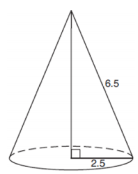mycollegehive
As shown in the diagram below, the radius of a cone is 2.5 c...
JMAP math geometry0As shown in the diagram below, the radius of a cone is 2.5 cm and its slant height is 6.5 cm.How many cubic centimeters are in the volume of the cone?

1) 12.5π

2) 13.5π

3) 30.0π

4) 37.5π

1.2K viewsShareFollowUniversity of Benin Nigeria
23 June 2020University of Lagos Nigeria
12 August 20200Volume of a cone, V = 1/3 πr2h

where r is the radius and h is the vertical height.

The vertical height can be found using pythagorean's theorem:

hyp is the slant height

opp is the vertical height

Thus, 6.52 = opp2 + 2.52

subtract 2.52 from both sides

opp2 = 6.52 - 2.52

opp2 = 36

Take square root of both sides,

opp = 6

Therefore, Vertical height = 6

V = 1/3 π(2.5)2(6)

V = 12.5π

Ans 1Share

### Groups

How to insert math formulas/equations### Related Tags

JMAP

14 followers

143 questionsmath

44 followers

1303 questionsgeometry

17 followers

99 questions